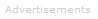# Definition of Mass

What is Mass?

Mass is a measure of the amount of matter in an object. Mass is usually measured in grams (g) or kilograms (kg).

Mass measures the quantity of matter regardless of both its location in the universe and the gravitational force applied to it. An object's mass is constant in all circumstances; contrast this with its weight, a force that depends on gravity.

Your mass on the earth and the moon are identical. Your weight on the moon is about one-sixth of your weight on the earth.

Rest Mass

To be more precise about mass, we need to mention the concept of rest mass, also known as intrinsic mass. It is actually an object's rest mass that is constant in all circumstances. Albert Einstein showed that mass actually increases with speed, although the effect is only significant at speeds that are substantial fractions of the speed of light.

Mass Conservation

One of the fundamental laws of chemistry is that of mass conservation. The mass of products emerging from a reaction is always identical to the mass of products that entered the reaction.

Is Mass Conservation True?

Chemical reactions obey the Einstein equation E = mc2 every bit as much as nuclear reactions do. So is the law of mass conservation actually true?Search the Dictionary for More Terms